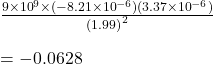## Suppose two point charges, Q1 = +3.37 x 10^-6 C and Q2 = -8.21 x 10^-6 C, are separated by a distance d = 1.99 m. Using

Question

Suppose two point charges, Q1 = +3.37 x 10^-6 C and Q2 = -8.21 x 10^-6 C, are separated by a distance d = 1.99 m.

Using Coulomb’s Law, find the attractive force between the charges.

A)
F = 0.0626 N

B)
F = 0.0313 N

C)
F = -0.0626 N

D)
F = -0.0313 N

in progress 0
5 months 2021-08-09T14:13:27+00:00 1 Answers 6 views 0